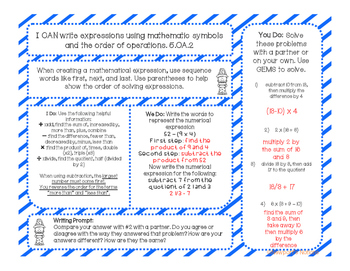5th Grade Math Interactive Notebook Unit 1Subject
Resource Type
Common Core Standards
Product Rating
4.0
3 Ratings
File Type

PDF (Acrobat) Document File

7 MB|70 pages
Share
Product Description
Interactive Notebooks provide students with a way to organize their thoughts as they learn. They also act as educational portfolios for teachers to monitor and track student performance. Interactive Notebooks are a great resource for student learning and they provide neat spaces for students to keep their learning over the course of a year in one place.

This packet includes:
-13 Common Core aligned interactive lessons with I CAN statements for each standard, a writing prompt, and practice problems in an "I Do, We Do, You Do" format
-a word problem for each standard with helpful notes
-answer sheets for all the problems included
-vocabulary cards for the unit
-multiple strategies for each standard
-teacher sheets for your own interactive notebook to record which students are struggling or need a challenge
-suggestions for remedial and challenging needs
-a place to keep data about the students in your class specific to each lesson
-essential question for each standard

Topics covered include:
Topics covered include:
~Lesson 1a: PEMDAS and/or GEMS
~Lesson 2a: using parentheses, brackets, and braces
~Lesson 3a: writing expressions using mathematics symbols
~Lesson 1b: place value
~Lesson 2b: multiplying by powers of 10
~Lesson 3b: dividing by powers of 10
~Lesson 4b: multiplying multi-digit whole numbers using area model
~Lesson 5b: multiplying multi-digit whole numbers using partial product
~Lesson 6b: multiplying multi-digit whole numbers using lattice
~Lesson 7b: multiplying multi-digit whole numbers using the traditional algorithm
~Lesson 8b: dividing multi-digit whole numbers using partial product
~Lesson 9b: dividing multi-digit whole numbers using the popcorn method
~Lesson 10b:dividing multi-digit whole numbers using long division

If you liked this product, check out the 5th Grade Math Interactive Notebook Unit 2
If you liked this product, check out the 5th Grade Math Interactive Notebook Unit 3
Total Pages
70 pages